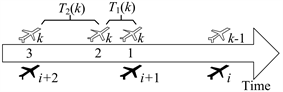﻿ 基于排队论模型的战时机场运行容量评估

# 基于排队论模型的战时机场运行容量评估Evaluation of Airdrome Operating Capacity in Wartime Based on Queuing Theory Model

Abstract: Military airdrome is an important support for the attack and defense of air force under the condi-tion of joint operations. The size of its capacity can improve the intensity of military aircraft, reduce the time-base interval between continuous aircraft flows, and provide further reference for joint operations planning. This paper uses queuing model of wartime military aircraft take-off, landing and single runway theoretical calculation, the operational capacity is derived for the military airfield runway per unit time, can hold the largest aircraft operation, on the basis of theoretical research combined with example analysis, adjustment and supplement of wartime military airdrome to provide the reference suggestion.

1. 引言

2. 战时机场运行容量计算

N——系统的最大容量；

$\lambda$ ——单位时间内机场系统平均着陆进近或起飞离场的平均到达数，即飞机平均到达率；

$\mu$ ——单位时间内机场跑道系统平均能保障的全起降飞机数，即机场平均保障率；

t——机场跑道的平均全起降保障时间，与 $\mu$ 互为倒数，即单位时间内机场跑道所保障的飞机起降均值数。

$\rho =\lambda t$ (1)

${p}_{0}=\frac{1-\rho }{1-{\rho }^{N+1}},\rho \ne 1$ (2)

${P}_{n}=\frac{1-\rho }{1-{\rho }^{N+1}}{\rho }^{n},n\le N$ (3)

${L}_{s}=\frac{\rho }{1-\rho }-\frac{\left(N+1\right){\rho }^{\left(N+1\right)}}{1-{\rho }^{\left(N+1\right)}},\rho \ne 1$ (4)

${W}_{s}=\frac{{L}_{s}}{\mu \left(1-{P}_{0}\right)}$ (5)

3. 战时机场全起飞运行容量计算

${\rho }_{d}={\lambda }_{d}{t}_{d}$ (6)

${p}_{0}{}_{d}=\frac{1-{\rho }_{d}}{1-{\rho }_{d}^{N+1}},{\rho }_{d}\ne 1$ (7)

${P}_{n}{}_{d}=\frac{1-{\rho }_{d}}{1-{\rho }_{d}^{{N}_{d}+1}}{\rho }_{d}^{n},n\le {N}_{d}$ (8)

${L}_{sd}=\frac{{\rho }_{d}}{1-{\rho }_{d}}-\frac{\left({N}_{d}+1\right){\rho }_{d}^{\left({N}_{d}+1\right)}}{1-{\rho }_{d}^{\left({N}_{d}+1\right)}},{\rho }_{d}\ne 1$ (9)

${W}_{s}{}_{d}=\frac{{L}_{sd}}{{\mu }_{d}\left(1-{P}_{0d}\right)}$ (10)

${t}_{d}$ ——跑道平均起飞保障占用时间。

${\lambda }_{d}$ ——飞机平均起飞率。

${\mu }_{d}$ ——跑道平均起飞保障率。

${W}_{q}{}_{d}={W}_{s}{}_{d}-{t}_{d}=\frac{\frac{{\rho }_{d}}{1-{\rho }_{d}}-\frac{\left({N}_{d}+1\right){\rho }_{d}^{\left({N}_{d}+1\right)}}{1-{\rho }_{d}^{\left({N}_{d}+1\right)}}-\left(1-\frac{1-{\rho }_{d}}{1-{\rho }_{d}^{\left({N}_{d}+1\right)}}\right)}{{\lambda }_{d}\left(1-\frac{1-{\rho }_{d}}{1-{\rho }_{d}^{\left({N}_{d}+1\right)}}{\rho }_{d}^{{N}_{d}}\right)}\le {t}_{d\mathrm{max}}$ (11)

$\frac{{\rho }_{d}^{\left({N}_{d}+1\right)}-{\rho }_{d}^{2}}{{\rho }_{d}-1}+\left(\frac{{t}_{d\mathrm{max}}}{{t}_{d}}-{N}_{d}\right){\rho }_{d}^{{N}_{d}}\le \frac{{t}_{d\mathrm{max}}}{{t}_{d}}$ (12)

$f\left({N}_{d}\right)=\frac{{\rho }_{d}^{\left({N}_{d}+1\right)}-{\rho }_{d}^{2}}{{\rho }_{d}-1}+\left(\frac{{t}_{d\mathrm{max}}}{{t}_{d}}-{N}_{d}\right){\rho }_{d}^{{N}_{d}}-\frac{{t}_{d\mathrm{max}}}{{t}_{d}}$ (13)

${N}_{d}=\frac{{t}_{d\mathrm{max}}}{{t}_{d}}-\frac{1}{\mathrm{ln}{\rho }_{d}}-\frac{{\rho }_{d}}{1-{\rho }_{d}}$ (14)

4. 战时机场全着陆运行容量计算

${\rho }_{a}={\lambda }_{a}{t}_{a}$ (15)

${P}_{0a}=\frac{1-{\rho }_{a}}{1-{\rho }_{a}^{{N}_{a}+1}},{\rho }_{a}\ne 1$ (16)

${P}_{n}{}_{a}=\frac{1-{\rho }_{a}}{1-{\rho }_{a}^{{N}_{a}+1}}{\rho }_{a}^{n},n\le {N}_{a}$ (17)

${L}_{sa}=\frac{{\rho }_{a}}{1-{\rho }_{a}}-\frac{\left({N}_{a}+1\right){\rho }_{a}^{\left({N}_{a}+1\right)}}{1-{\rho }_{a}^{\left({N}_{a}+1\right)}},{\rho }_{a}\ne 1$ (18)

${W}_{s}{}_{a}=\frac{{L}_{sa}}{{\mu }_{a}\left(1-{P}_{0}{}_{a}\right)}$ (19)

${W}_{q}{}_{a}={W}_{sa}-\frac{1}{{\mu }_{a}}=\frac{\frac{{\rho }_{a}}{1-{\rho }_{a}}-\frac{\left({N}_{a}+1\right){\rho }_{a}^{\left({N}_{a}+1\right)}}{1-{\rho }_{a}^{\left({N}_{a}+1\right)}}-\left(1-\frac{1-{\rho }_{a}}{1-{\rho }_{a}^{\left({N}_{a}+1\right)}}\right)}{{\lambda }_{a}\left(1-\frac{1-{\rho }_{a}}{1-{\rho }_{a}^{\left({N}_{a}+1\right)}}{\rho }_{a}^{{N}_{a}}\right)}$ (20)

${t}_{a}$ ——机场跑道保障着陆平均占用时间。

${\lambda }_{a}$ ——飞机平均着陆率。

${\mu }_{a}$ ——跑道平均着陆保障率。

${W}_{q}{}_{a}={W}_{s}{}_{a}-\frac{1}{{\mu }_{a}}=\frac{\frac{{\rho }_{a}}{1-{\rho }_{a}}-\frac{\left({N}_{a}+1\right){\rho }_{a}^{\left({N}_{a}+1\right)}}{1-{\rho }_{a}^{\left({N}_{a}+1\right)}}-\left(1-\frac{1-{\rho }_{a}}{1-{\rho }_{a}^{\left({N}_{a}+1\right)}}\right)}{{\lambda }_{a}\left(1-\frac{1-{\rho }_{a}}{1-{\rho }_{a}^{\left({N}_{a}+1\right)}}{\rho }_{a}^{{N}_{a}}\right)}\le {t}_{a\mathrm{max}}$ (21)

$\frac{{\rho }_{a}^{\left({N}_{a}+1\right)}-{\rho }_{a}^{2}}{{\rho }_{a}-1}+\left(\frac{{t}_{a\mathrm{max}}}{{t}_{a}}-{N}_{a}\right){\rho }_{a}^{{N}_{a}}\le \frac{{t}_{a\mathrm{max}}}{{t}_{a}}$ (22)

$f\left({N}_{a}\right)=\frac{{\rho }_{a}^{\left({N}_{a}+1\right)}-{\rho }_{a}^{2}}{{\rho }_{a}-1}+\left(\frac{{t}_{a\mathrm{max}}}{{t}_{a}}-{N}_{a}\right){\rho }_{a}^{{N}_{a}}-\frac{{t}_{a\mathrm{max}}}{{t}_{a}}$ (23)

${N}_{a}=\frac{{t}_{a\mathrm{max}}}{{t}_{a}}-\frac{1}{\mathrm{ln}{\rho }_{a}}-\frac{{\rho }_{a}}{1-{\rho }_{a}}$ (24)

5. 战时机场混合起降运行容量计算注：1) 轴上方为起飞航空器，轴下方为着陆航空器；2) 1、2、3代表起飞航空器3种插缝放行情形在时间轴上的位置。

Figure 1. Schematic diagram of slotting release

$\left\{\begin{array}{l}{W}_{d}={{W}^{\prime }}_{qd}+{W}_{T\left(k\right)}\\ {{W}^{\prime }}_{qd}=\frac{{\lambda }_{d}{{t}^{\prime }}_{d}^{2}}{1-{\lambda }_{d}{{t}^{\prime }}_{d}}\\ {W}_{T\left(k\right)}=\frac{{g}_{a}{{t}^{\prime }}_{a}^{2}}{1-{\lambda }_{a}{{t}^{\prime }}_{a}}\end{array}$ (25)

${W}_{d}={{W}^{\prime }}_{qd}+{W}_{T\left(k\right)}=\frac{{\lambda }_{d}{{t}^{\prime }}_{d}^{2}}{1-{\lambda }_{d}{{t}^{\prime }}_{d}}+\frac{{g}_{a}{{t}^{\prime }}_{a}^{2}}{1-{\lambda }_{a}{{t}^{\prime }}_{a}}$ 求导取极小值时，得

${\lambda }_{d}=\frac{{W}_{d}}{2{{t}^{\prime }}_{d}{W}_{d}+2{{t}^{\prime }}_{d}^{2}}+\frac{{W}_{d}+{{t}^{\prime }}_{d}-{g}_{a}\cdot {t}_{a}^{2}}{8{{t}^{\prime }}_{d}{t}_{a}^{2}\left({W}_{d}+{{t}^{\prime }}_{d}\right)}$ (26)

${{N}^{\prime }}_{d}={\lambda }_{d}$ (27)

$N={{N}^{\prime }}_{d}+{N}_{a}$ (28)

6. 实例分析Table 1. Aircraft takeoff and landing observation data in the exercise flightTable 2. Data of average runway time occupied by takeoff and landing

1) 计算跑道起飞容量。

${N}_{d}=\frac{{t}_{d\mathrm{max}}}{{t}_{d}}-\frac{1}{\mathrm{ln}{\rho }_{d}}-\frac{{\rho }_{d}}{1-{\rho }_{d}}$

2) 计算跑道着陆容量。

${N}_{a}=\frac{{t}_{a\mathrm{max}}}{{t}_{a}}-\frac{1}{\mathrm{ln}{\rho }_{a}}-\frac{{\rho }_{a}}{1-{\rho }_{a}}$

3) 计算跑道混合起降容量。

$\left\{\begin{array}{l}{g}_{a}=P\left\{\frac{1}{{\lambda }_{a}}>{{t}^{\prime }}_{a}\right\}\\ {W}_{d}={{W}^{\prime }}_{qd}+{W}_{T\left(k\right)}=\frac{{\lambda }_{d}{{t}^{\prime }}_{d}^{2}}{1-{\lambda }_{d}{{t}^{\prime }}_{d}}+\frac{{g}_{a}{{t}^{\prime }}_{a}^{2}}{1-{\lambda }_{a}{{t}^{\prime }}_{a}}\\ {\lambda }_{d}=\frac{{W}_{d}}{2{{t}^{\prime }}_{d}{W}_{d}+2{{t}^{\prime }}_{d}^{2}}+\frac{{W}_{d}+{{t}^{\prime }}_{d}-{g}_{a}\cdot {t}_{a}^{2}}{8{{t}^{\prime }}_{d}{t}_{a}^{2}\left({W}_{d}+{{t}^{\prime }}_{d}\right)}\end{array}$

4) 设跑道运行容量为N，则

$N=\mathrm{max}\left\{{{N}^{\prime }}_{d}+{N}_{a},{N}_{a},{N}_{d}\right\}$

7. 结束语

 孔航, 胡明华, 姚逸, 裔田园. 不同运行模式下跑道容量评估技术研究[J]. 航空计算技术, 2017.

 唐应辉, 唐小我. 排队论-基础与分析技术[M]. 北京: 科学出版社, 2006.

 Graham, R. (2015) RECAT-EU European Wake Turbulence Categorisation and Separation Minima on Approach and Departure. Eurocontrol, Europe.

 Federal Aviation Administration (2013) SAFO 12007. Re-Categorization (RECAT) of FAA Wake Turbulence Separation Categories at Specific Airports. Federal Aviation Administration, Washington, DC.

 王声. 战时机场保障概论[M]. 北京: 空军后勤部机场管理部, 2005.

 王莉莉, 陈云翔. 考虑机场容量的作战飞机调配保障模型[J]. 电光与控制, 2010, 01-0054-03.

 潘卫军, 冉斌, 吴郑源, 朱新平 . 尾流间隔分类标准综述[J]. 中国民航飞行学院学报, 2019(2): 54-57.

 王声. 战时机场保障概论[M]. 北京: 空军后勤部机场管理部, 2005.

Top